Chapter 9 Other Graphs

Graphs in this chapter can be very useful, but don’t fit in easily within the other chapters.

9.1 3-D Scatterplot

The ggplot2 package and its extensions can’t create a 3-D plot. However, you can create a 3-D scatterplot with the scatterplot3d function in the scatterplot3d package.

Let’s say that we want to plot automobile mileage vs. engine displacement vs. car weight using the data in the mtcars dataframe.

# basic 3-D scatterplot
library(scatterplot3d)
with(mtcars, {
scatterplot3d(x = disp,
y = wt,
z = mpg,
main="3-D Scatterplot Example 1")
})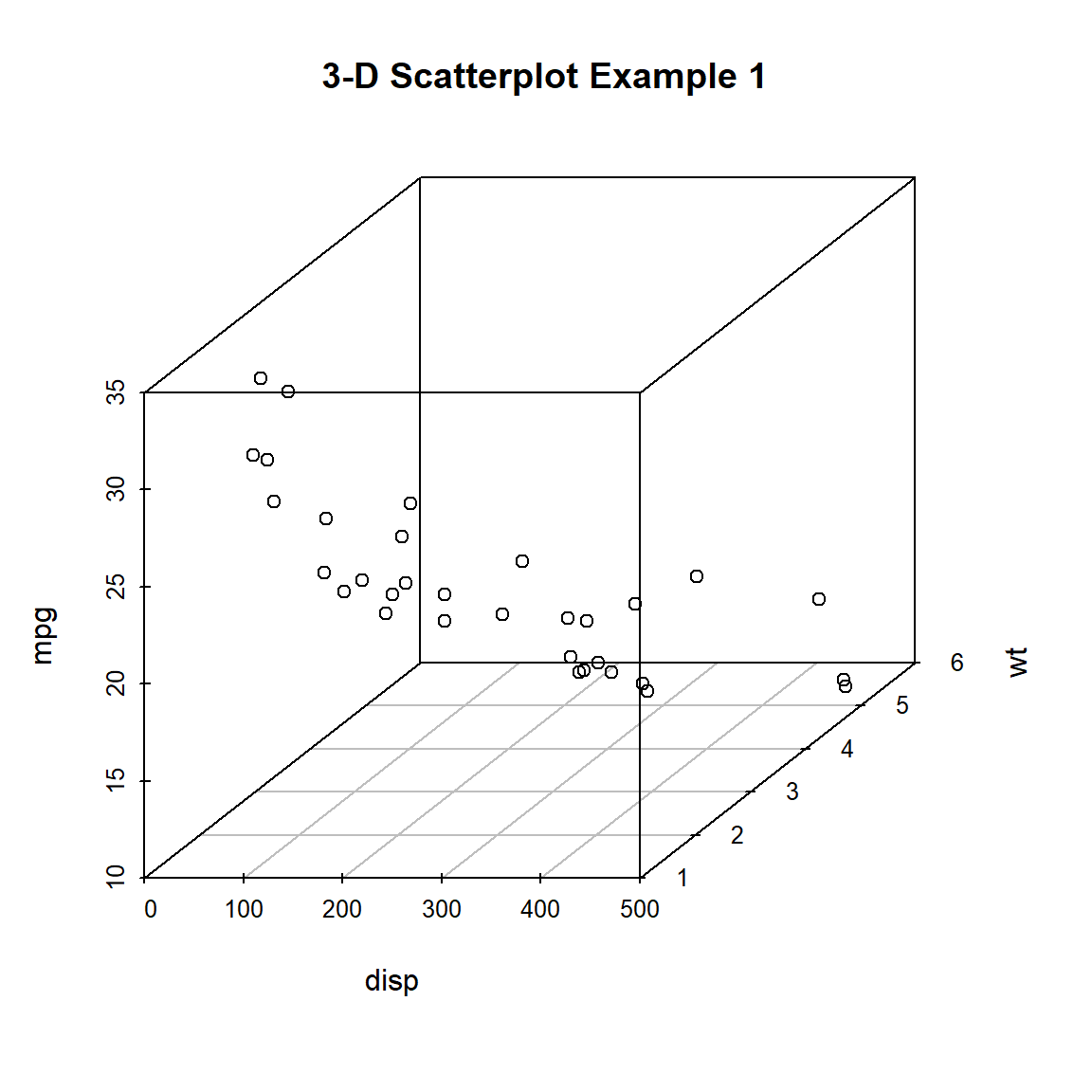Figure 9.1: Basic 3-D scatterplot

Now lets, modify the graph by replacing the points with filled blue circles, add drop lines to the x-y plane, and create more meaningful labels.

library(scatterplot3d)
with(mtcars, {
scatterplot3d(x = disp,
y = wt,
z = mpg,
# filled blue circles
color="blue",
pch=19,
# lines to the horizontal plane
type = "h",
main = "3-D Scatterplot Example 2",
xlab = "Displacement (cu. in.)",
ylab = "Weight (lb/1000)",
zlab = "Miles/(US) Gallon")
})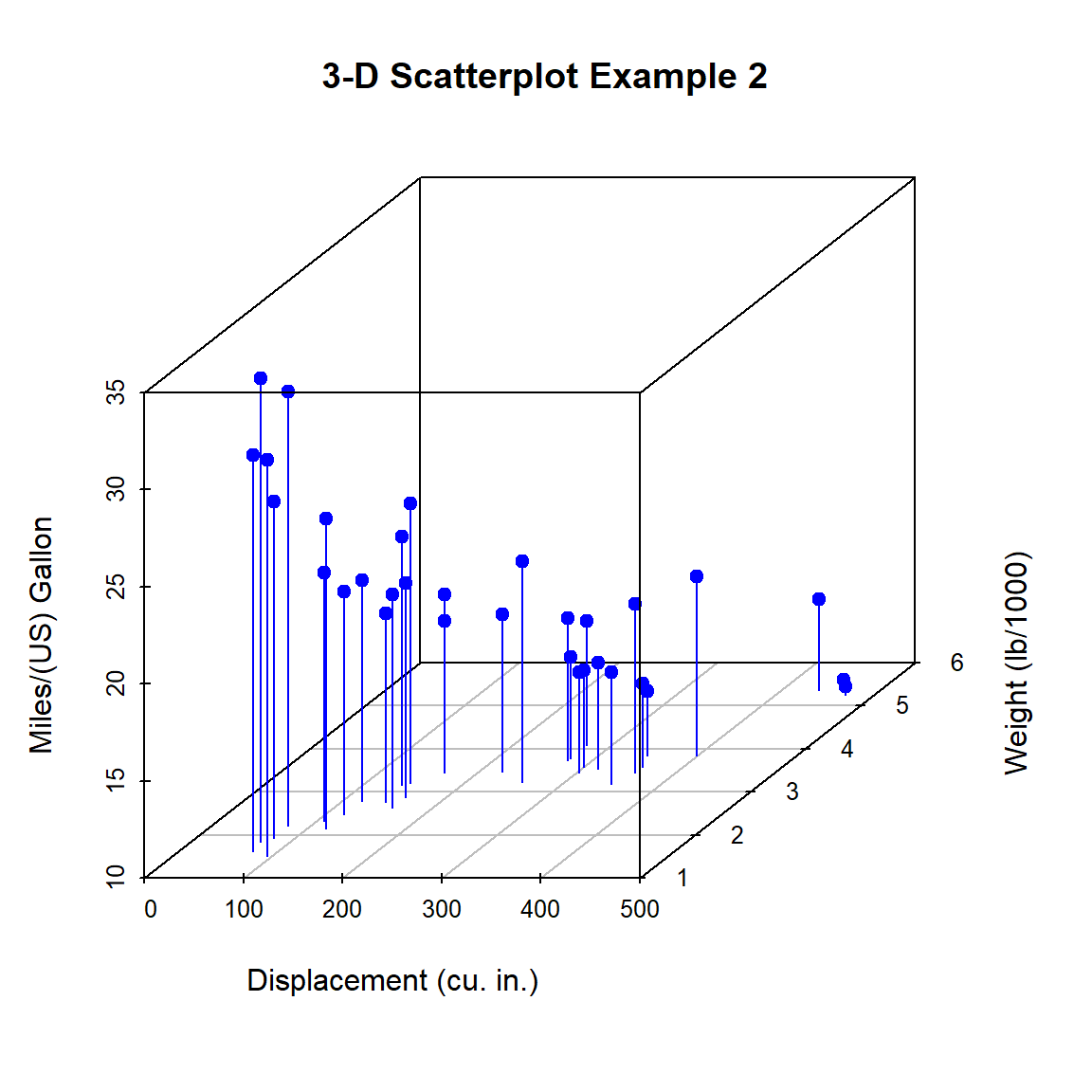Figure 9.2: 3-D scatterplot with vertical lines

Next, let’s label the points. We can do this by saving the results of the scatterplot3d function to an object, using the xyz.convert function to convert coordinates from 3-D (x, y, z) to 2D-projections (x, y), and apply the text function to add labels to the graph.

library(scatterplot3d)
with(mtcars, {
s3d <- scatterplot3d(
x = disp,
y = wt,
z = mpg,
color = "blue",
pch = 19,
type = "h",
main = "3-D Scatterplot Example 3",
xlab = "Displacement (cu. in.)",
ylab = "Weight (lb/1000)",
zlab = "Miles/(US) Gallon")

# convert 3-D coords to 2D projection
s3d.coords <- s3d$xyz.convert(disp, wt, mpg) # plot text with 50% shrink and place to right of points text(s3d.coords$x,
s3d.coords$y, labels = row.names(mtcars), cex = .5, pos = 4) })Figure 9.3: 3-D scatterplot with vertical lines and point labels Almost there. As a final step, we will add information on the number of cylinders in each car. To do this, we’ll add a column to the mtcars dataframe indicating the color for each point. For good measure, we will shorten the y-axis, change the drop lines to dashed lines, and add a legend. library(scatterplot3d) # create column indicating point color mtcars$pcolor[mtcars$cyl == 4] <- "red" mtcars$pcolor[mtcars$cyl == 6] <- "blue" mtcars$pcolor[mtcars$cyl == 8] <- "darkgreen" with(mtcars, { s3d <- scatterplot3d( x = disp, y = wt, z = mpg, color = pcolor, pch = 19, type = "h", lty.hplot = 2, scale.y = .75, main = "3-D Scatterplot Example 4", xlab = "Displacement (cu. in.)", ylab = "Weight (lb/1000)", zlab = "Miles/(US) Gallon") s3d.coords <- s3d$xyz.convert(disp, wt, mpg)
text(s3d.coords$x, s3d.coords$y,
labels = row.names(mtcars),
pos = 4,
cex = .5)

legend(#location
"topleft",
inset=.05,
# suppress legend box, shrink text 50%
bty="n",
cex=.5,
title="Number of Cylinders",
c("4", "6", "8"),
fill=c("red", "blue", "darkgreen"))
})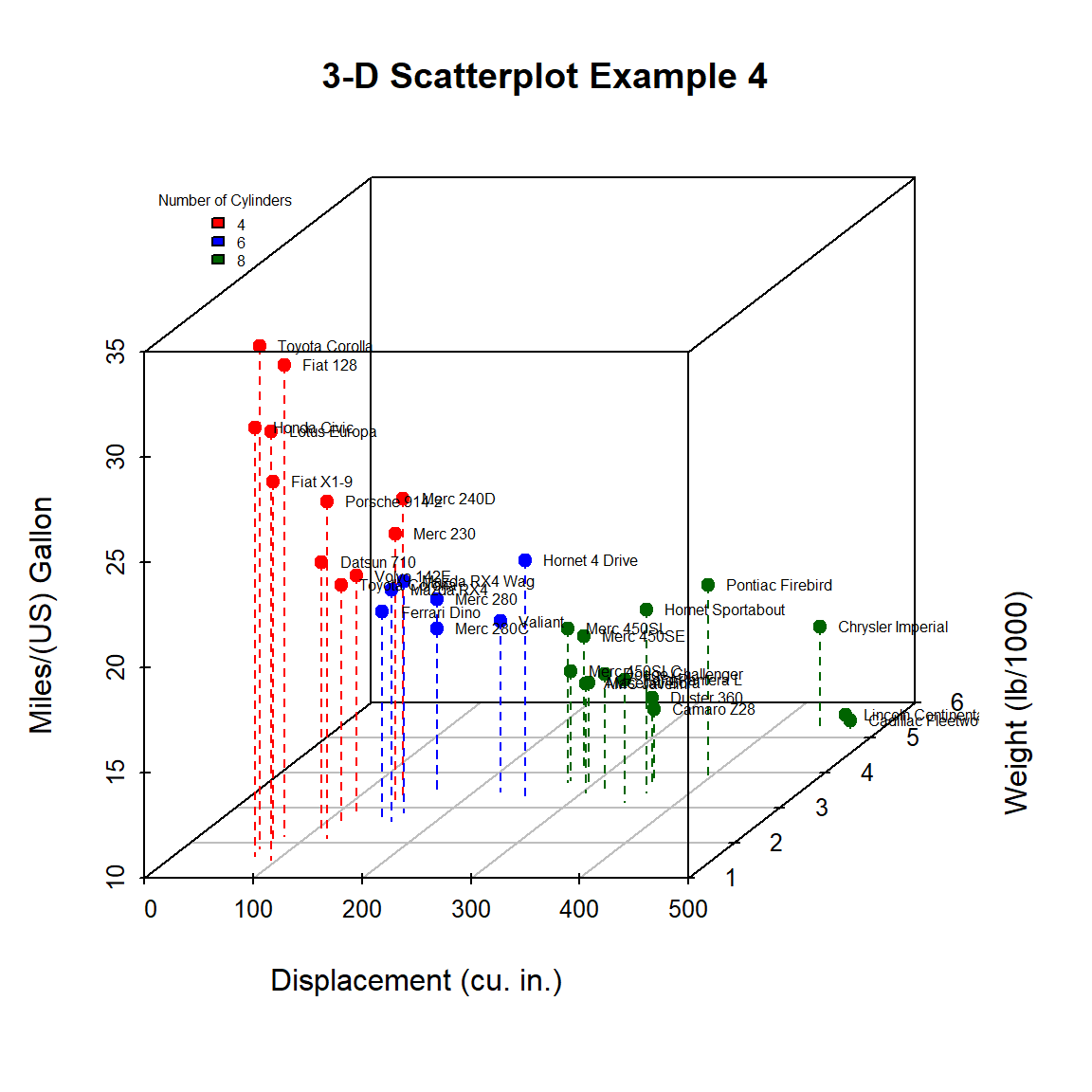Figure 9.4: 3-D scatterplot with vertical lines and point labels and legend

We can easily see that the car with the highest mileage (Toyota Corolla) has low engine displacement, low weight, and 4 cylinders.

9.2 Biplots

A biplot is a specialized graph that attempts to represent the relationship between observations, between variables, and between observations and variables, in a low (usually two) dimensional space.

It’s easiest to see how this works with an example. Let’s create a biplot for the mtcars dataset, using the fviz_pca function from the factoextra package.

# create a biplot
data(mtcars)

# fit a principal components model
fit <- prcomp(x = mtcars,
center = TRUE,
scale = TRUE)

# plot the results
library(factoextra)
fviz_pca(fit,
repel = TRUE,
labelsize = 3) +
theme_bw() +
labs(title = "Biplot of mtcars data")Figure 9.5: Basic biplot

The fviz_pca function produces a ggplot2 graph.

Dim1 and Dim2 are the first two principal components - linear combinations of the original p variables.

$PC_{1} = \beta_{10} +\beta_{11}x_{1} + \beta_{12}x_{2} + \beta_{13}x_{3} + \dots + \beta_{1p}x_{p}$ $PC_{2} = \beta_{20} +\beta_{21}x_{1} + \beta_{22}x_{2} + \beta_{23}x_{3} + \dots + \beta_{2p}x_{p}$

The weights of these linear combinations ($$\beta_{ij}s$$) are chosen to maximize the variance accounted for in the original variables. Additionally, the principal components (PCs) are constrained to be uncorrelated with each other.

In this graph, the first PC accounts for 60% of the variability in the original data. The second PC accounts for 24%. Together, they account for 84% of the variability in the original p = 11 variables.

As you can see, both the observations (cars) and variables (car characteristics) are plotted in the same graph.

• Points represent observations. Smaller distances between points suggest similar values on the original set of variables. For example, the Toyota Corolla and Honda Civic are similar to each other, as are the Chrysler Imperial and Liconln Continental. However, the Toyota Corolla is very different from the Lincoln Continental.
• The vectors (arrows) represent variables. The angle between vectors are proportional to the correlation between the variables. Smaller angles indicate stronger correlations. For example, gear and am are positively correlated, gear and qsec are uncorrelated (90 degree angle), and am and wt are negatively correlated (angle greater then 90 degrees).
• The observations that are are farthest along the direction of a variable’s vector, have the highest values on that variable. For example, the Toyoto Corolla and Honda Civic have higher values on mpg. The Toyota Corona has a higher qsec. The Duster 360 has more cylinders.

Care must be taken in interpreting biplots. They are only accurate when the percentage of variance accounted for is high. Always check your conclusion with the original data.

9.3 Bubble charts

A bubble chart is basically just a scatterplot where the point size is proportional to the values of a third quantitative variable.

Using the mtcars dataset, let’s plot car weight vs. mileage and use point size to represent horsepower.

# create a bubble plot
data(mtcars)
library(ggplot2)
ggplot(mtcars,
aes(x = wt, y = mpg, size = hp)) +
geom_point()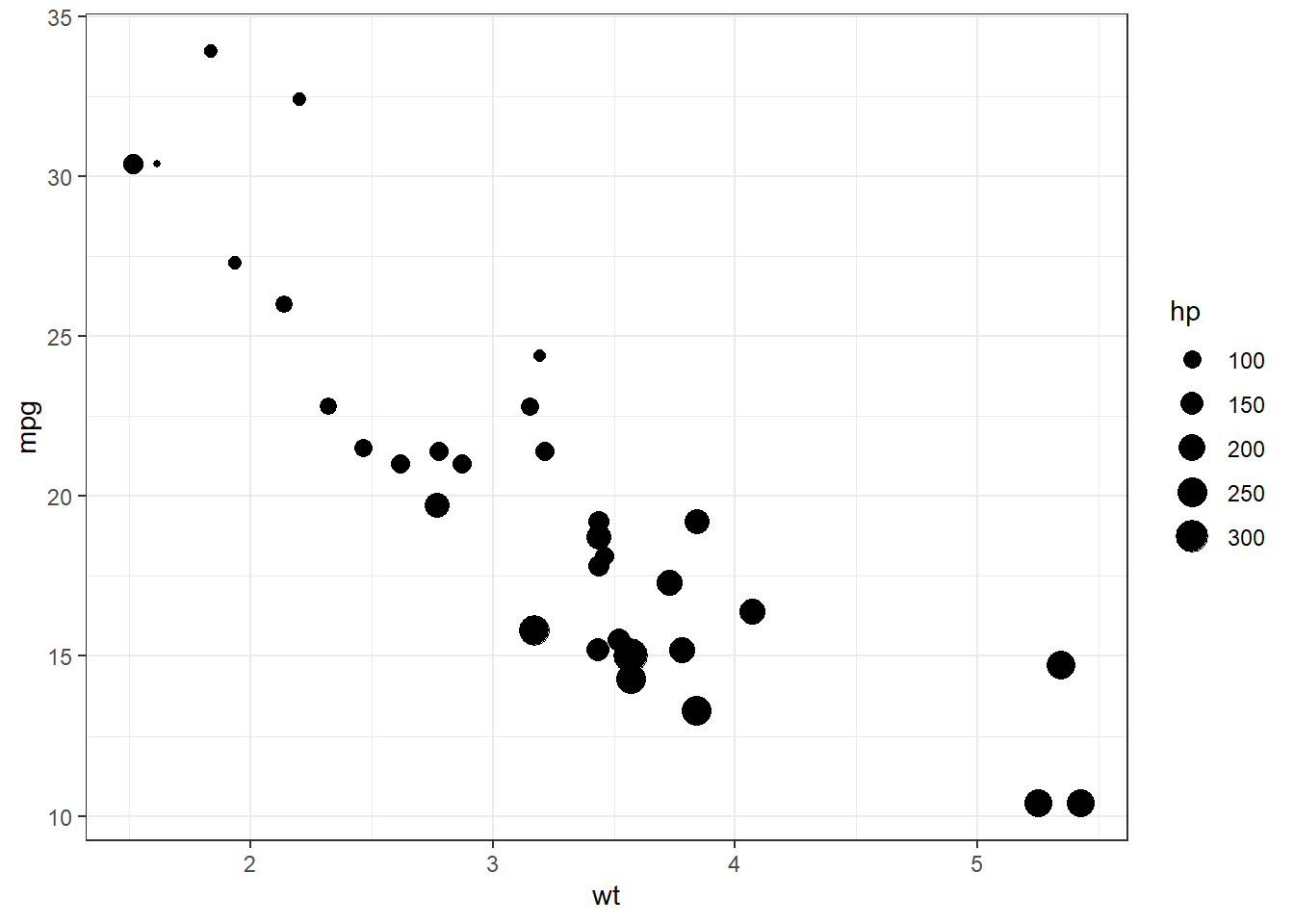Figure 9.6: Basic bubble plot

We can improve the default appearance by increasing the size of the bubbles, choosing a different point shape and color, and adding some transparency.

# create a bubble plot with modifications
ggplot(mtcars,
aes(x = wt, y = mpg, size = hp)) +
geom_point(alpha = .5,
fill="cornflowerblue",
color="black",
shape=21) +
scale_size_continuous(range = c(1, 14)) +
labs(title = "Auto mileage by weight and horsepower",
subtitle = "Motor Trend US Magazine (1973-74 models)",
x = "Weight (1000 lbs)",
y = "Miles/(US) gallon",
size = "Gross horsepower")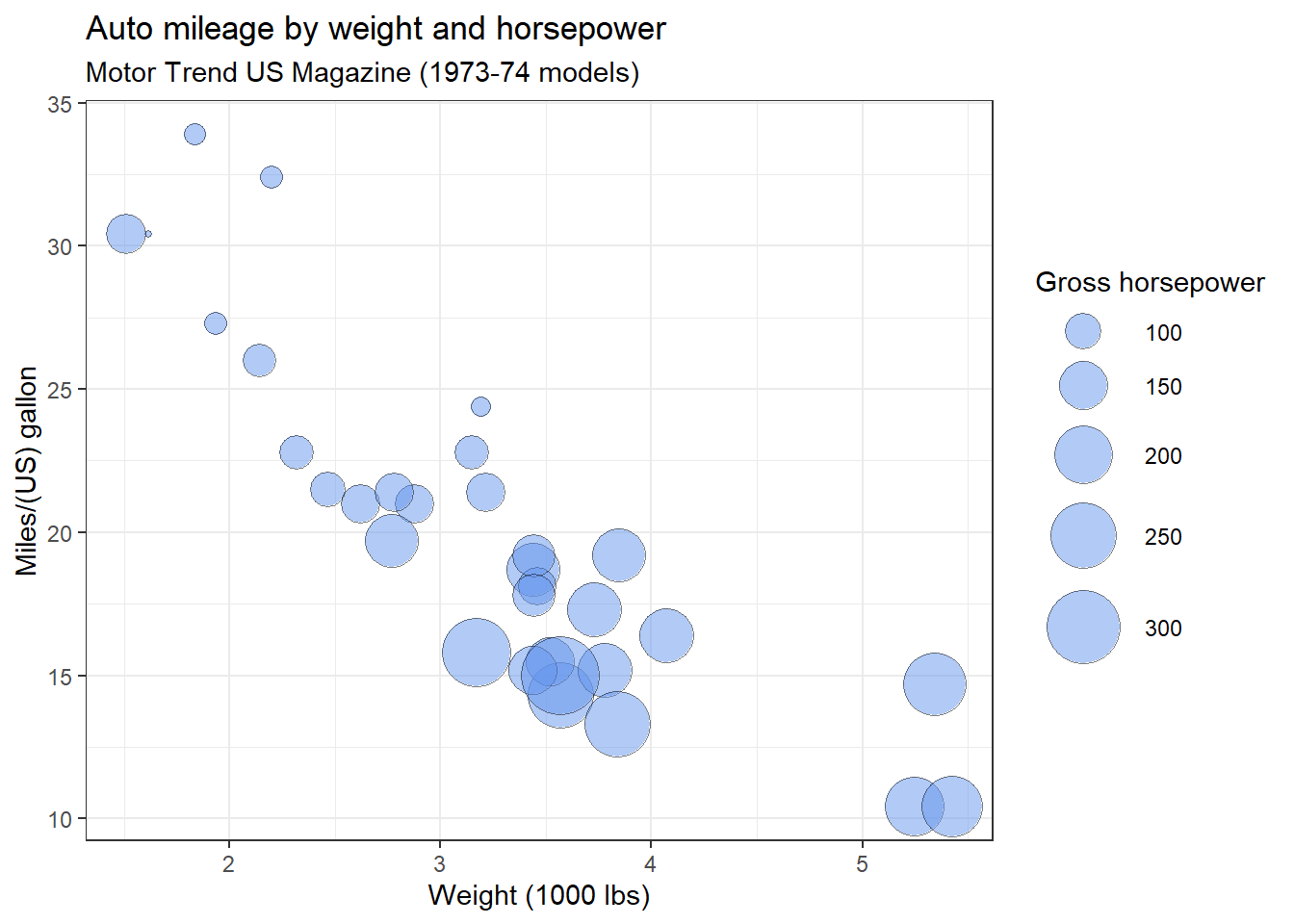Figure 9.7: Bubble plot with modifications

The range parameter in the scale_size_continuous function specifies the minimum and maximum size of the plotting symbol. The default is range = c(1, 6).

The shape option in the geom_point function specifies an circle with a border color and fill color.

Clearly, miles per gallon decreases with increased car weight and horsepower. However, there is one car with low weight, high horsepower, and high gas mileage. Going back to the data, it’s the Lotus Europa.

Bubble charts are controversial for the same reason that pie charts are controversial. People are better at judging length than volume. However, they are quite popular.

9.4 Flow diagrams

A flow diagram represents a set of dynamic relationships. It usually captures the physical or metaphorical flow of people, materials, communications, or objects through a set of nodes in a network.

9.4.1 Sankey diagrams

In a Sankey diagram, the width of the line between two nodes is proportional to the flow amount. We’ll demonstrate this with UK energy forecast data. The data contain energy production and consumption forecasts for the year 2050.

Building the graph requires two data frames, one containing node names and the second containing the links between the nodes and the amount of the flow between them.

# input data (data frames nodes and links)

# view nodes data frame
## # A tibble: 6 x 1
##   name
##   <chr>
## 1 Agricultural 'waste'
## 2 Bio-conversion
## 3 Liquid
## 4 Losses
## 5 Solid
## 6 Gas
## # A tibble: 6 x 3
##   source target   value
##    <int>  <int>   <dbl>
## 1      0      1 125.
## 2      1      2   0.597
## 3      1      3  26.9
## 4      1      4 280.
## 5      1      5  81.1
## 6      6      2  35.0

We’ll build the diagram using the sankeyNetwork function in the networkD3 package.

# create Sankey diagram
library(networkD3)
Nodes = nodes,
Source = "source",
Target = "target",
Value = "value",
NodeID = "name",
units = "TWh", # optional units name for popups
fontSize = 12,
nodeWidth = 30)

Figure 9.8: Sankey diagram

Energy supplies are on the left and energy demands are on the right. Follow the flow from left to right. Notice that the graph is interactive (assuming you are viewing it on a web page). Try highlighting nodes and dragging them to new positions.

Sankey diagrams created with the networkD3 package are not ggplot2 graphs. Therefore, they can not be modified with ggplot2 functions.

9.4.2 Alluvial diagrams

Alluvial diagrams are a subset of Sankey diagrams, and are more rigidly defined. A discussion of the differences can be found here.

When examining the relationship among categorical variables, alluvial diagrams can serve as alternatives to mosaic plots. In an alluvial diagram, blocks represent clusters of observations, and stream fields between the blocks represent changes to the composition of the clusters over time.

They can also be used when time is not a factor. As an example, let’s diagram the survival of Titanic passengers, using the Titanic dataset.

Alluvial diagrams are created with ggalluvial package, generating ggplot2 graphs.

# input data

# summarize data
library(dplyr)
titanic_table <- titanic %>%
group_by(Class, Sex, Survived) %>%
count()

titanic_table$Survived <- factor(titanic_table$Survived,
levels = c("Yes", "No"))

## # A tibble: 6 x 4
## # Groups:   Class, Sex, Survived 
##   Class Sex    Survived     n
##   <chr> <chr>  <fct>    <int>
## 1 1st   Female No           4
## 2 1st   Female Yes        141
## 3 1st   Male   No         118
## 4 1st   Male   Yes         62
## 5 2nd   Female No          13
## 6 2nd   Female Yes         93
# create alluvial diagram
library(ggplot2)
library(ggalluvial)

ggplot(titanic_table,
aes(axis1 = Class,
axis2 = Survived,
y = n)) +
geom_alluvium(aes(fill = Sex)) +
geom_stratum() +
geom_text(stat = "stratum",
label.strata = TRUE) +
scale_x_discrete(limits = c("Class", "Survived"),
expand = c(.1, .1)) +
labs(title = "Titanic data",
subtitle = "stratified by class, sex, and survival",
y = "Frequency") +
theme_minimal()Figure 9.9: Basic alluvial diagram

Start at a node on the left and follow the stream field to the right. The height of the blocks represent the proportion of observations in that cluster and the height of the stream field represents the proportion of observations contained in both blocks they connect.

For example, most crew are male and do not survive. A much larger percent of 1st class females survive, than 1st class males.

Here is an alternative visualization. Survived becomes an axis and Class becomes the fill color. Colors are chosen from the viridis palette. Additionally, the legend is suppressed.

# create alternative alluvial diagram
library(ggplot2)
library(ggalluvial)
ggplot(titanic_table,
aes(axis1 = Class,
axis2 = Sex,
axis3 = Survived,
y = n)) +
geom_alluvium(aes(fill = Class)) +
geom_stratum() +
geom_text(stat = "stratum",
label.strata = TRUE) +
scale_x_discrete(limits = c("Class", "Sex", "Survived"),
expand = c(.1, .1)) +
scale_fill_viridis_d() +
labs(title = "Titanic data",
subtitle = "stratified by class, sex, and survival",
y = "Frequency") +
theme_minimal() +
theme(legend.position = "none")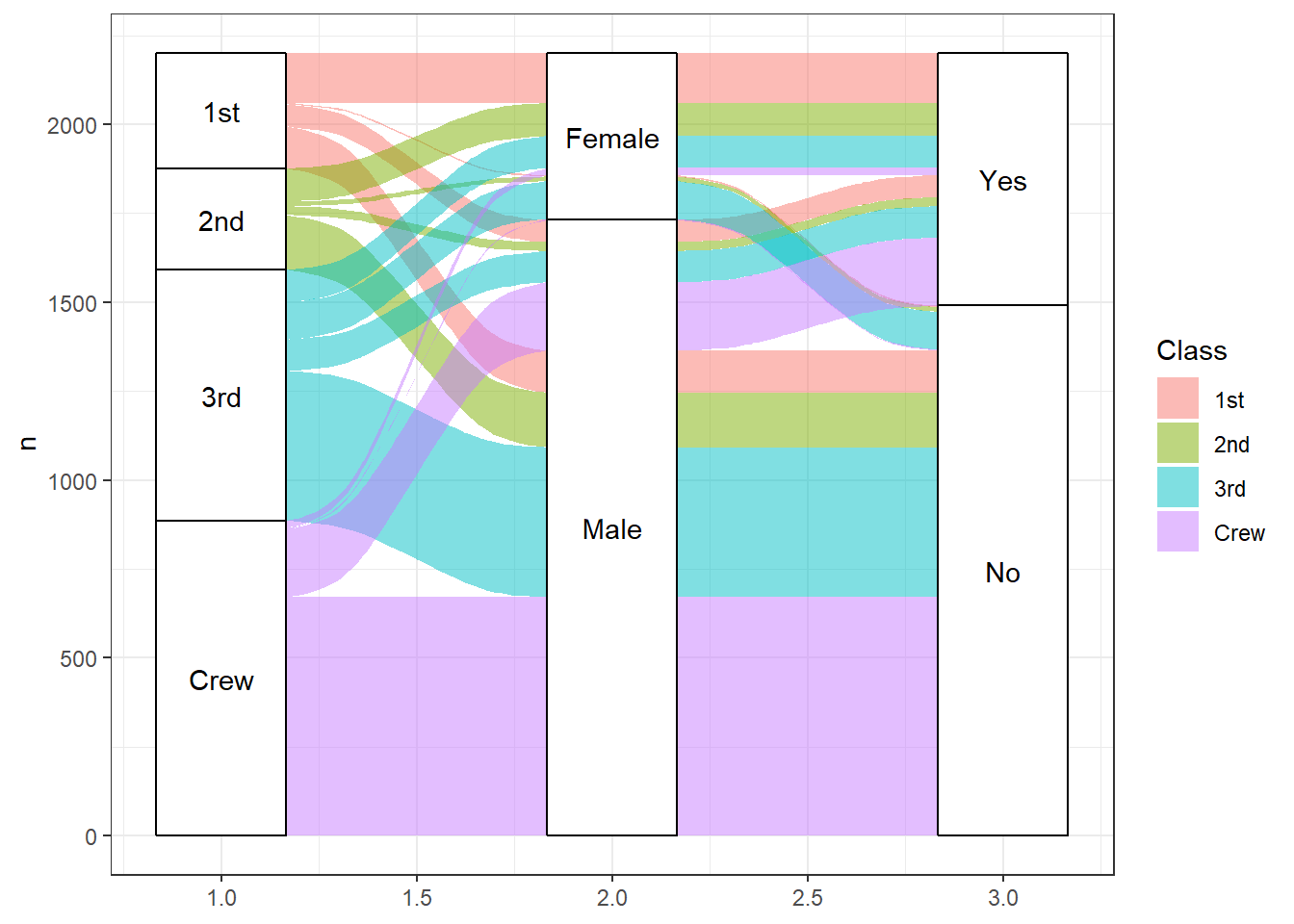Figure 9.10: Alternative alluvial diagram

I think that this version is a bit easier to follow.

See the ggalluvial website for additional details.

9.5 Heatmaps

A heatmap displays a set of data using colored tiles for each variable value within each observation. There are many varieties of heatmaps. Although base R comes with a heatmap function, we’ll use the more powerful superheat package (I love these names).

First, let’s create a heatmap for the mtcars dataset that come with base R. The mtcars dataset contains information on 32 cars measured on 11 variables.

# create a heatmap
data(mtcars)
library(superheat)
superheat(mtcars, scale = TRUE)Figure 9.11: Basic heatmap

The scale = TRUE options standardizes the columns to a mean of zero and standard deviation of one. Looking at the graph, we can see that the Merc 230 has a quarter mile time (qsec) the is well above average (bright yellow). The Lotus Europa has a weight is well below average (dark blue).

We can use clustering to sort the rows and/or columns. In the next example, we’ll sort the rows so that cars that are similar appear near each other. We will also adjust the text and label sizes.

# sorted heat map
superheat(mtcars,
scale = TRUE,
left.label.text.size=3,
bottom.label.text.size=3,
bottom.label.size = .05,
row.dendrogram = TRUE )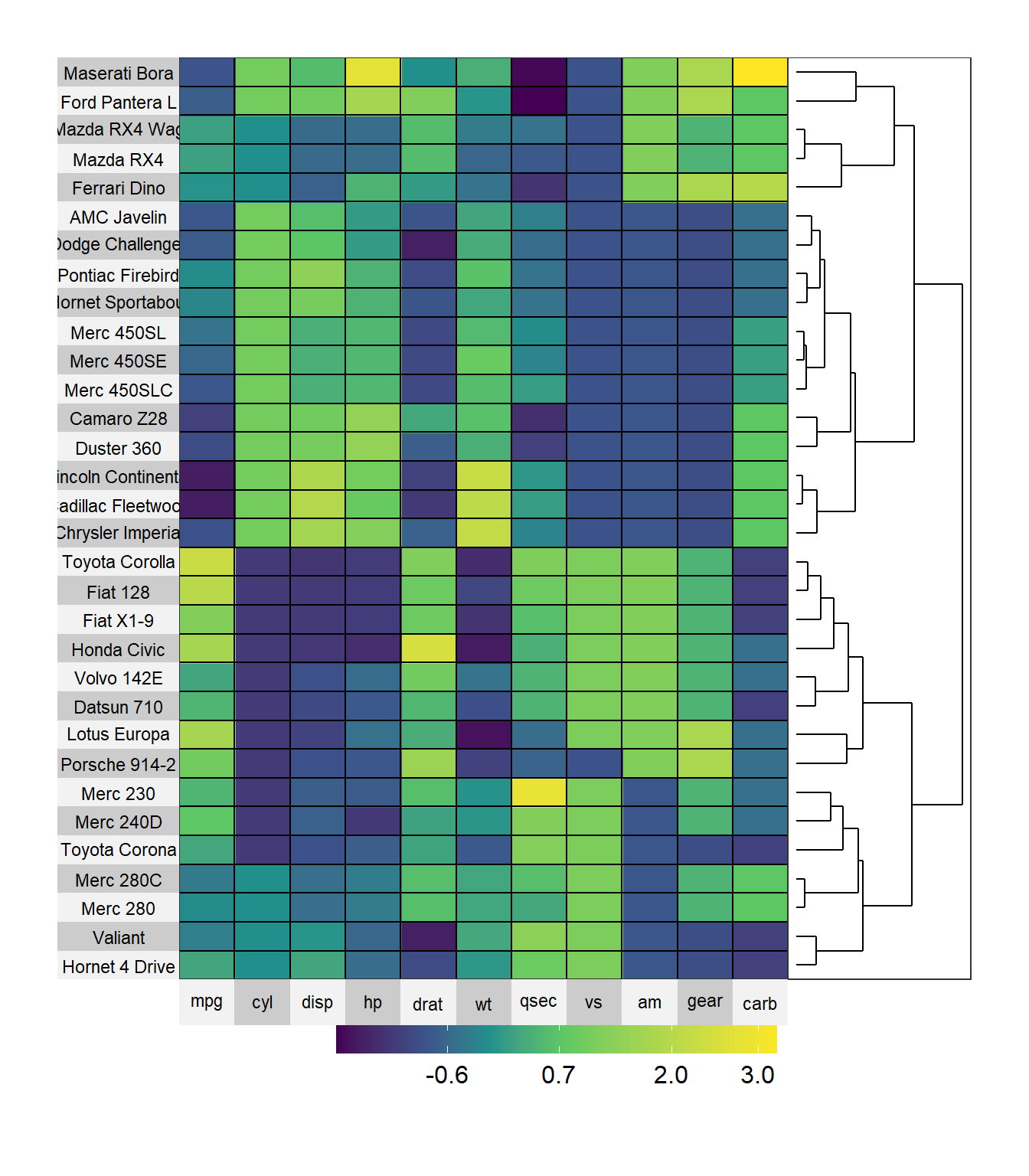Figure 9.12: Sorted heatmap

Here we can see that the Toyota Corolla and Fiat 128 have similar characteristics. The Lincoln Continental and Cadillac Fleetwood also have similar characteristics.

The superheat function requires that the data be in particular format. Specifically

• the data most be all numeric
• the row names are used to label the left axis. If the desired labels are in a column variable, the variable must be converted to row names (more on this below)
• missing values are allowed

Let’s use a heatmap to display changes in life expectancies over time for Asian countries. The data come from the gapminder dataset.

Since the data is in long format, we first have to convert to wide format. Then we need to ensure that it is a data frame and convert the variable country into row names. Finally, we’ll sort the data by 2007 life expectancy. While we are at it, let’s change the color scheme.

# create heatmap for gapminder data (Asia)
library(tidyr)
library(dplyr)

data(gapminder, package="gapminder")

# subset Asian countries
asia <- gapminder %>%
filter(continent == "Asia") %>%
select(year, country, lifeExp)

# convert to long to wide format

# save country as row names
plotdata <- as.data.frame(plotdata)
row.names(plotdata) <- plotdata$country plotdata$country <- NULL

# row order
sort.order <- order(plotdata\$"2007")

# color scheme
library(RColorBrewer)
colors <- rev(brewer.pal(5, "Blues"))

# create the heat map
superheat(plotdata,
scale = FALSE,
left.label.text.size=3,
bottom.label.text.size=3,
bottom.label.size = .05,
heat.pal = colors,
order.rows = sort.order,
title = "Life Expectancy in Asia")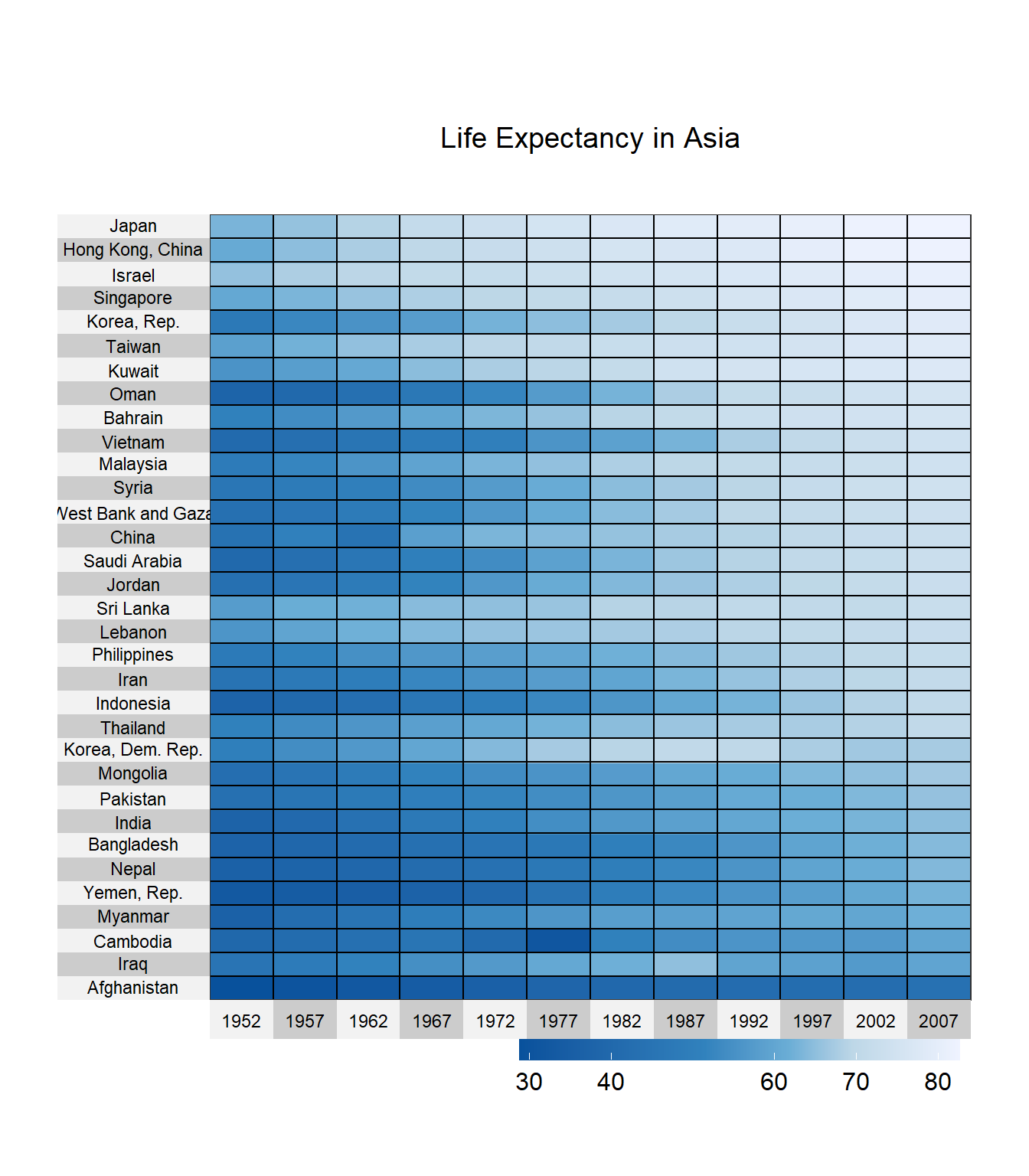Figure 9.13: Heatmap for time series

Japan, Hong Kong, and Israel have the highest life expectancies. South Korea was doing well in the 80s but has lost some ground. Life expectancy in Cambodia took a sharp hit in 1977.

To see what you can do with heat maps, see the extensive superheat vignette.

A radar chart (also called a spider or star chart) displays one or more groups or observations on three or more quantitative variables.

In the example below, we’ll compare dogs, pigs, and cows in terms of body size, brain size, and sleep characteristics (total sleep time, length of sleep cycle, and amount of REM sleep). The data come from the Mammal Sleep dataset.

Radar charts can be created with ggradar function in the ggradar package. Unfortunately, the package in not available on CRAN, so we have to install it from Github.

install.packages("devtools")

Next, we have to put the data in a specific format:

• The first variable should be called group and contain the identifier for each observation
• The numeric variables have to be rescaled so that their values range from 0 to 1

# prepare data
data(msleep, package = "ggplot2")
library(scales)
library(dplyr)

plotdata <- msleep %>%
filter(name %in% c("Cow", "Dog", "Pig")) %>%
select(name, sleep_total, sleep_rem,
sleep_cycle, brainwt, bodywt) %>%
rename(group = name) %>%
mutate_at(vars(-group),
funs(rescale))
plotdata

grid.label.size = 4,
axis.label.size = 4,
group.point.size = 5,
group.line.width = 1.5,
legend.text.size= 10) +
labs(title = "Mammals, size, and sleep")In the previous chart, the mutate_at function rescales all variables except group. The various size options control the font sizes for the percent labels, variable names, point size, line width, and legend labels respectively.

We can see from the chart that, relatively speaking, cows have large brain and body weights, long sleep cycles, short total sleep time and little time in REM sleep. Dogs in comparison, have small body and brain weights, short sleep cycles, and a large total sleep time and time in REM sleep (The obvious conclusion is that I want to be a dog - but with a bigger brain).

9.7 Scatterplot matrix

A scatterplot matrix is a collection of scatterplots organized as a grid. It is similar to a correlation plot but instead of displaying correlations, displays the underlying data.

You can create a scatterplot matrix using the ggpairs function in the GGally package.

We can illustrate its use by examining the relationships between mammal size and sleep characteristics. The data come from the msleep dataset that ships with ggplot2. Brain weight and body weight are highly skewed (think mouse and elephant) so we’ll transform them to log brain weight and log body weight before creating the graph.

library(GGally)

# prepare data
data(msleep, package="ggplot2")
library(dplyr)
df <- msleep %>%
mutate(log_brainwt = log(brainwt),
log_bodywt = log(bodywt)) %>%
select(log_brainwt, log_bodywt, sleep_total, sleep_rem)

# create a scatterplot matrix
ggpairs(df)Figure 9.14: Scatterplot matrix

By default,

• the principal diagonal contains the kernel density charts for each variable.
• The cells below the principal diagonal contain the scatterplots represented by the intersection of the row and column variables. The variables across the top are the x-axes and the variables down the right side are the y-axes.
• The cells above the principal diagonal contain the correlation coefficients.

For example, as brain weight increases, total sleep time and time in REM sleep decrease.

The graph can be modified by creating custom functions.

# custom function for density plot
my_density <- function(data, mapping, ...){
ggplot(data = data, mapping = mapping) +
geom_density(alpha = 0.5,
fill = "cornflowerblue", ...)
}

# custom function for scatterplot
my_scatter <- function(data, mapping, ...){
ggplot(data = data, mapping = mapping) +
geom_point(alpha = 0.5,
color = "cornflowerblue") +
geom_smooth(method=lm,
se=FALSE, ...)
}

# create scatterplot matrix
ggpairs(df,
lower=list(continuous = my_scatter),
diag = list(continuous = my_density)) +
labs(title = "Mammal size and sleep characteristics") +
theme_bw()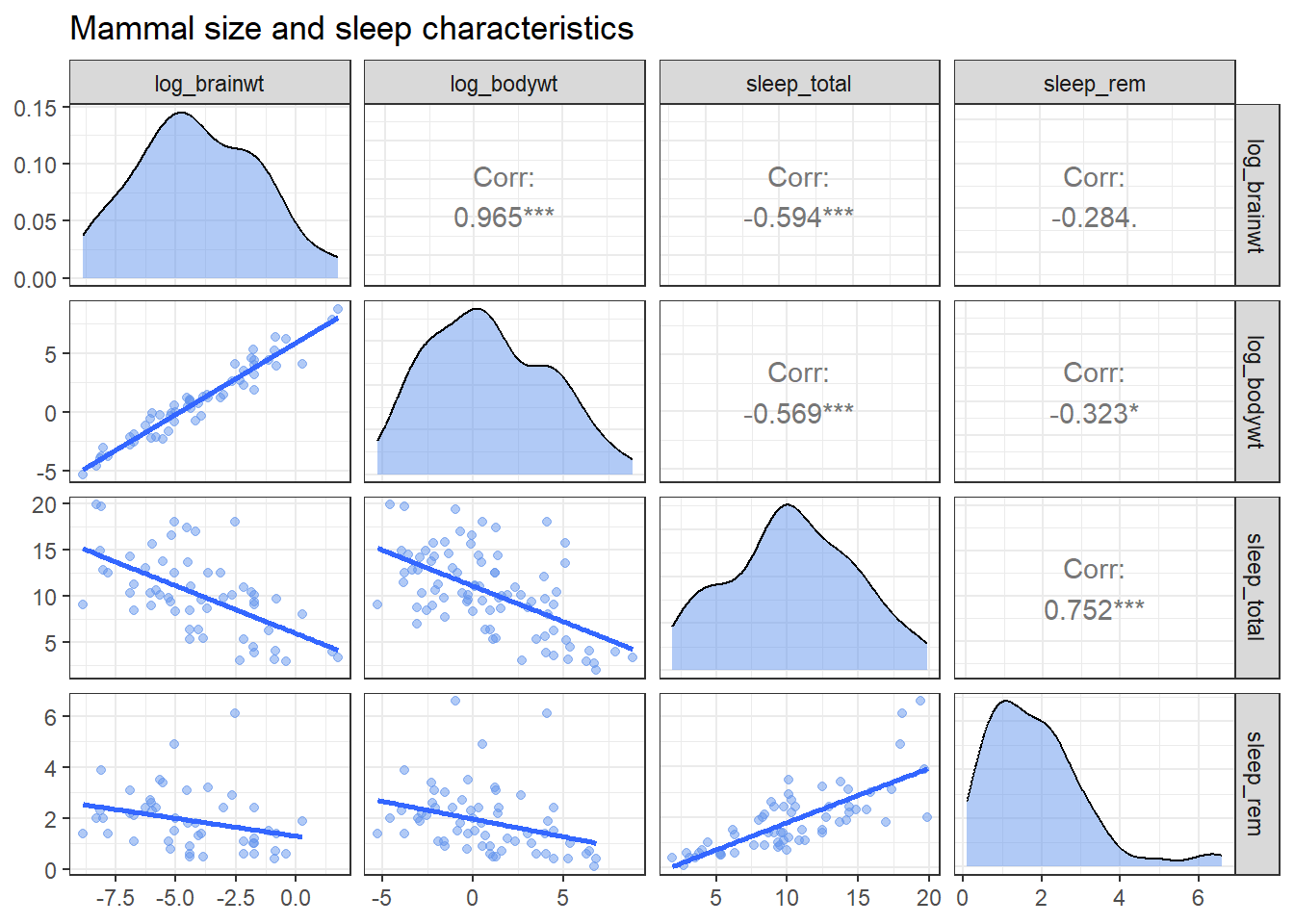Figure 9.15: Customized scatterplot matrix

Being able to write your own functions provides a great deal of flexibility. Additionally, since the resulting plot is a ggplot2 graph, addition functions can be added to alter the theme, title, labels, etc. See the help for more details.

9.8 Waterfall charts

A waterfall chart illustrates the cumulative effect of a sequence of positive and negative values.

For example, we can plot the cumulative effect of revenue and expenses for a fictional company. First, let’s create a dataset

# create company income statement
category <- c("Sales", "Services", "Fixed Costs",
"Variable Costs", "Taxes")
amount <- c(101000, 52000, -23000, -15000, -10000)
income <- data.frame(category, amount)

Now we can visualize this with a waterfall chart, using the waterfall function in the waterfalls package.

# create waterfall chart
library(ggplot2)
library(waterfalls)
waterfall(income)Figure 9.16: Basic waterfall chart

We can also add a total (net) column. Since the result is a ggplot2 graph, we can use additional functions to customize the results.

# create waterfall chart with total column
waterfall(income,
calc_total=TRUE,
total_axis_text = "Net",
total_rect_text_color="black",
total_rect_color="goldenrod1") +
scale_y_continuous(label=scales::dollar) +
labs(title = "West Coast Profit and Loss",
subtitle = "Year 2017",
y="",
x="") +
theme_minimal()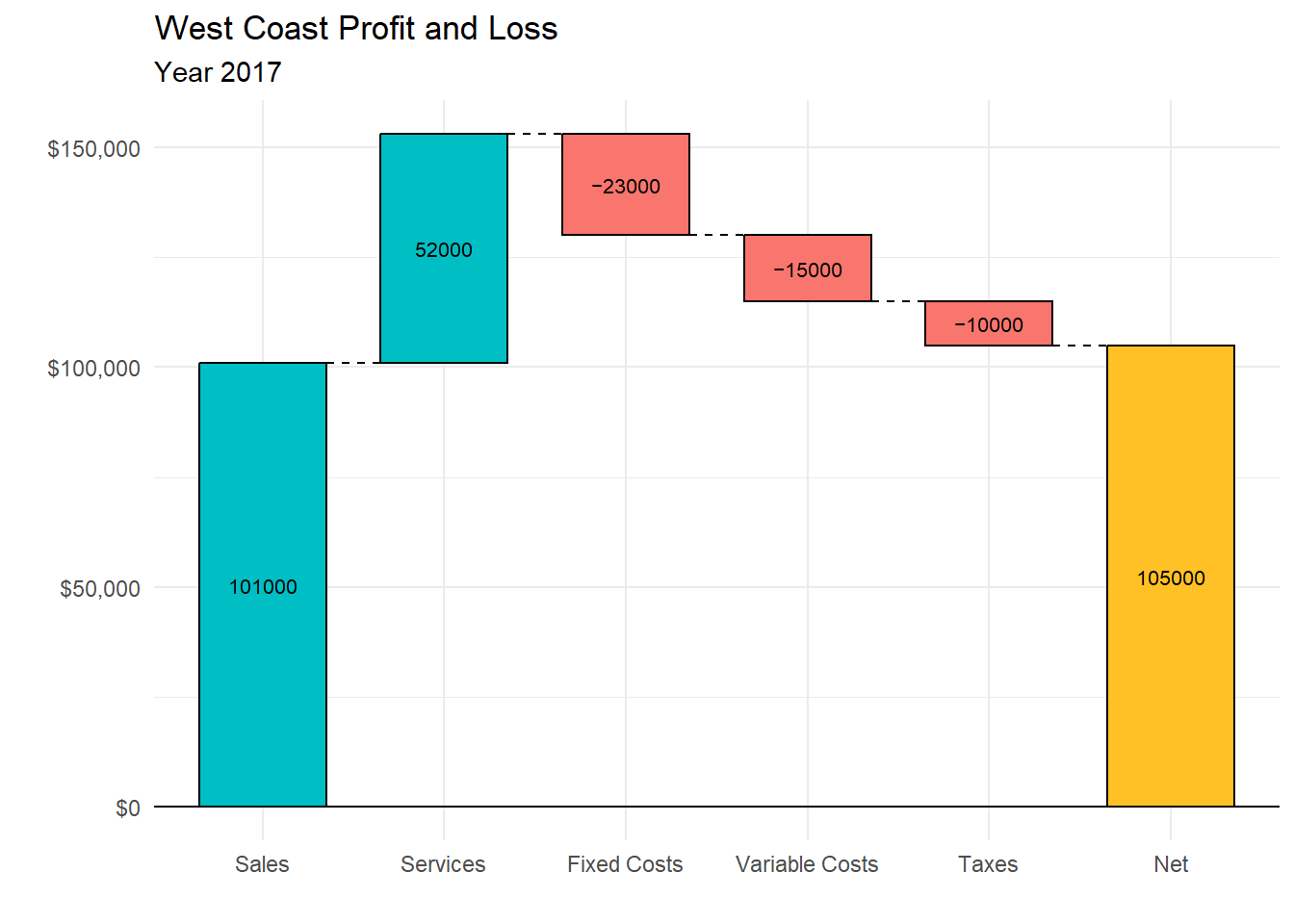Figure 9.17: Waterfall chart with total column

9.9 Word clouds

A word cloud (also called a tag cloud), is basically an infographic that indicates the frequency of words in a collection of text (e.g., tweets, a text document, a set of text documents). There is a very nice script produced by STHDA that will generate a word cloud directly from a text file.

To demonstrate, we’ll use President Kennedy’s Address during the Cuban Missile crisis.

To use the script, there are several packages you need to install first.

# install packages for text mining
install.packages(c("tm", "SnowballC",
"wordcloud", "RColorBrewer",
"RCurl", "XML")

Once the packages are installed, you can run the script on your text file.

# create a word cloud